This header plots the critical line of the Riemann Zeta Function.  A complete understanding wins a \$1,000,000 prize.
 . . .Main Links Orders Post Next Page Next + 10

Dissections of Convex Figures

Michael Reid has a site devoted to Rectifiable Polyominoes.  More polyomino rectangles are at Torsten Sillke's site.  For the Math Magic problem of the month, Erich Friedman asks about n-convex figures.  All of this has lead me to wonder about something I'll call Nontrivial Convexity.  The following three figures display nontrivial convexity.  The first two are by Ed Pegg Jr (me), the third is by Karl Scherer, courtesy of Michael Reid.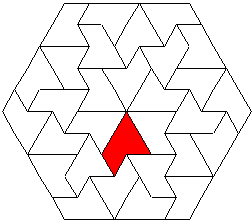In a convex figure, if you pick any two points, the points between them are also a part of the figure.  A figure without this property is nonconvex.If you can assemble convex figure from n copies of figure A , then figure A is n-convex.  Here are a few examples of what I consider Trivial Convexity.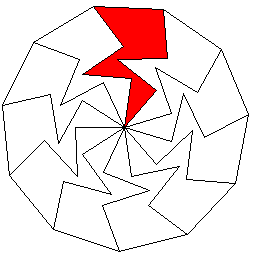Trivial examples display simple rotational or translational symmetry.  For more examples of this, visit Doris Schattschneider's Tiling site.  These trivial examples of attaining convexity are easy to find.

I'm interested in the Nontrivial examples, such as the following.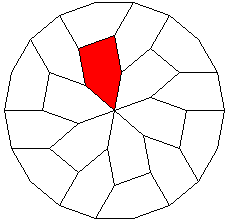The first shape was found by Michael Hirschhorn, the second by John Wallace (US Patent 4350341, Sep 21 1982), the third by William Rex Marshall (see Reid's site).  Reid's list of contains 10-vex (the y-pentomino), 18-vex, 24-vex, 28-vex, 50-vex (the figure above), 76-vex, 92-vex, 96-vex, 138-vex, 192-vex, 272-vex, and 420-vex polyomino diagrams.  These are the minimal numbers for each shape.  Most of these figures also have odd numbered convexities.  It is currently unknown whether there exists a polyomino with an odd minimal rectangle.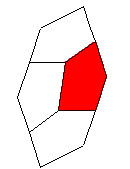Here is the same pentagon as above, demonstrating it is 9-vex, again.  The Spectrum for this shape is thus {1, 2, 9, 9, 14, 18} (Is that all).  I discovered this one.  The next two are by Michael Dowle.  I pulled them from his excellent lists gracing my Iamond page.  The rectifiable polyominoes are well studied, but the convexifiable iamonds (hexagonifiable?) are not.  How many of the enneiamonds are n-convex?  The last is the only other class of  n-vex convex pentagon I was able to find.  Are there more?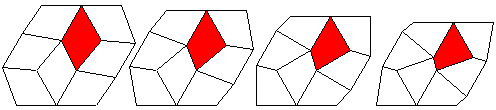There is a related class of quadrilaterals which are 8-vex.  I've made an interactive applet based on the above figures.  I'd love to see more examples of non-trivial n-vex figures. Send answers.